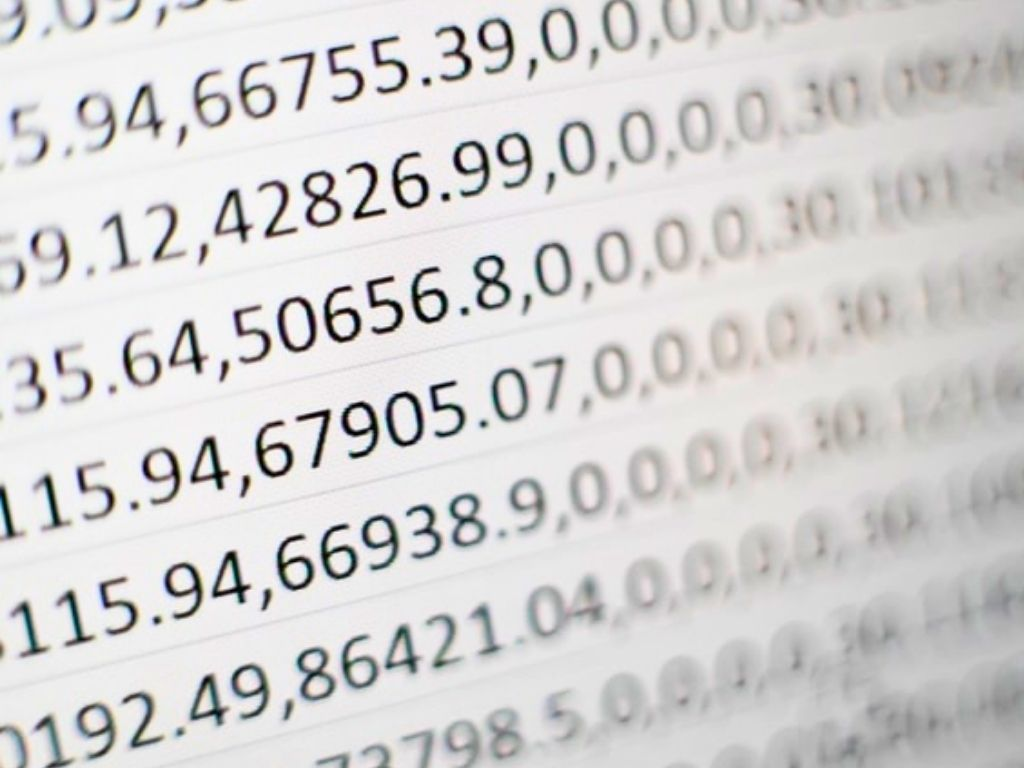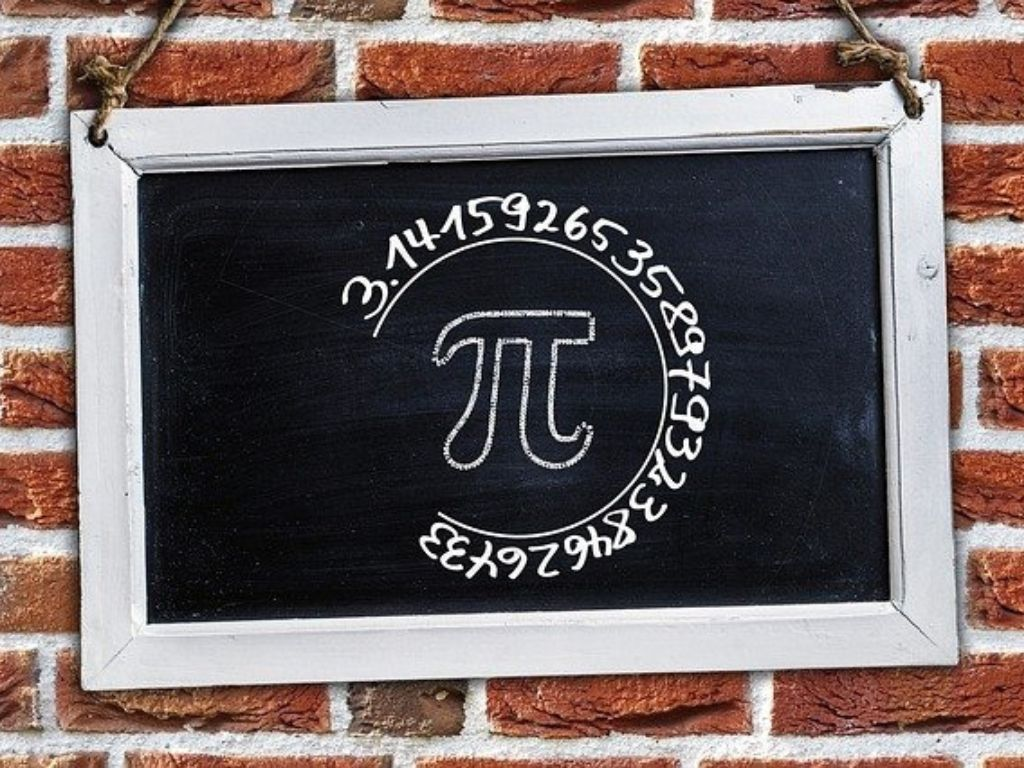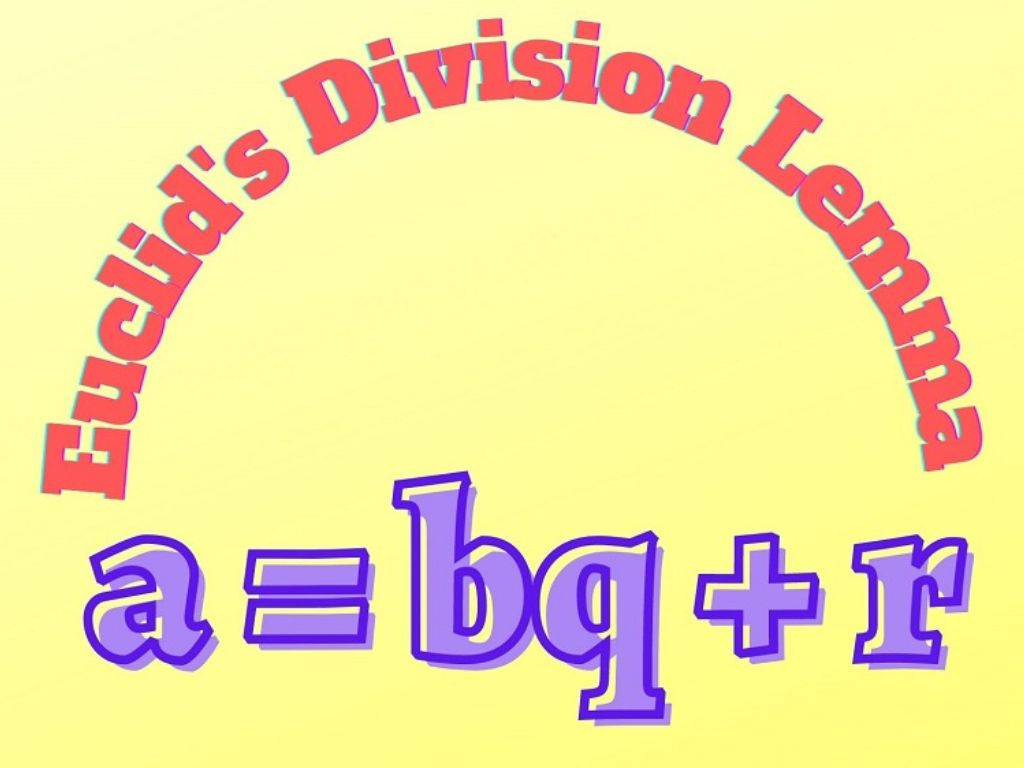# Number System Class 10th## Decimal Expansion of Rational Numbers Class 10th

Introduction We have already studied that the number which can be written in the form of p/q is called a Rational Number. Where p and q are integers and q ≠ 0. The decimal expansion of rational numbers is either a terminating decimal expansion or a non-terminating repeating (recurring) decimal expansion. In this section, we …## Irrational Numbers and Irrationality Class 10th

Introduction Irrational Numbers – The numbers that cannot be written in the form of p/q, are called Irrational Numbers. Where p and q are integers and q ≠ 0. Irrational numbers are denoted by T. Examples – √2, √3, √5, π, etc. In this section, we shall study the proof of the irrationality of an …## The Fundamental Theorem of Arithmetic Class 10th

Introduction The Fundamental Theorem of Arithmetic is related to Number System. We have already studied prime numbers and composite numbers. The numbers which are divisible by 1 or the number itself are called Prime Numbers and the numbers which are divisible by other numbers also are called Composite Numbers. The factors of a prime number …## Euclid’s Division Lemma Class 10th

Introduction Euclid’s Division Lemma is generally an algorithm that is derived by Greek Mathematician Euclid. This lemma is based on the Division of Real Numbers. We have already studied the division algorithm, in which if we divide a number by another number we get two numbers a quotient and a remainder (sometimes the remainder may …